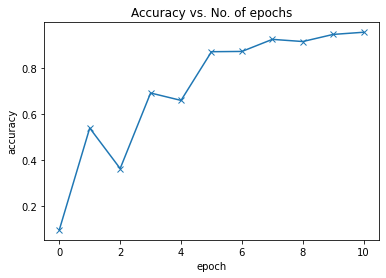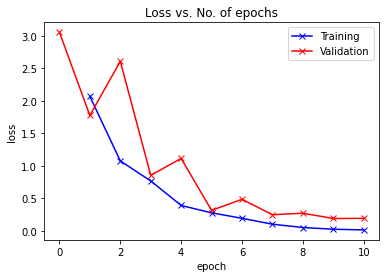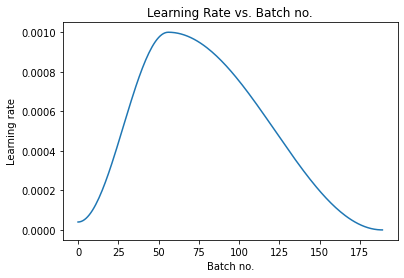Learn data science and machine learning by building real-world projects on Jovian

We are going to use the Free Spoken Digit Dataset (FSDD) to create a ResNet that identifies spoken digits.

The dataset consists of recordings of spoken digits in wav files at 8kHz. The recordings are trimmed so that they have near minimal silence at the beginnings and ends.

The dataset has 3,000 recordings from total of 6 speakers (50 of each digit per speaker) at the time of writing.

The audio data can be represented in many forms, like for example, as a time series vector, or as a spectrogram (image). However, we use Mel-frequency cepstral coefficients (MFCCs) as it has been found to be a better representation of sound for deep learning.

In :
``project_name='fsdd-audio-classification'``
In :
``````# To play audio files inside the notebook
from IPython.display import Audio``````

In :
``````# Download and extract the dataset and rename the folder to 'fsdd'
!wget https://github.com/Jakobovski/free-spoken-digit-dataset/archive/master.zip
!unzip -q master.zip
!rm -rf master.zip
!mv free-spoken-digit-dataset-master fsdd
``````
```--2020-12-27 08:19:09-- https://github.com/Jakobovski/free-spoken-digit-dataset/archive/master.zip Resolving github.com (github.com)... 140.82.113.3 Connecting to github.com (github.com)|140.82.113.3|:443... connected. HTTP request sent, awaiting response... 302 Found Location: https://codeload.github.com/Jakobovski/free-spoken-digit-dataset/zip/master [following] --2020-12-27 08:19:09-- https://codeload.github.com/Jakobovski/free-spoken-digit-dataset/zip/master Resolving codeload.github.com (codeload.github.com)... 140.82.112.10 Connecting to codeload.github.com (codeload.github.com)|140.82.112.10|:443... connected. HTTP request sent, awaiting response... 200 OK Length: unspecified [application/zip] Saving to: ‘master.zip’ master.zip [ <=> ] 15.66M 15.9MB/s in 1.0s 2020-12-27 08:19:10 (15.9 MB/s) - ‘master.zip’ saved  ```

Let's take a look at a few clips to familiarize ourselves with the data.

In :
``audio_dir = "./fsdd/recordings/"``
In :
``Audio(audio_dir + "1_george_0.wav")``
Out:
In :
``Audio(audio_dir + "6_jackson_0.wav")``
Out:
In :
``Audio(audio_dir + "0_theo_0.wav")``
Out:

## Processing the dataset and generating MFCCs

In :
``````import os
import librosa
import numpy as np
import h5py``````

The function `audio_to_mfcc()` loads the wav file into memory, scales it down to half the original size, and generates 20 MFCCs for every frame (of 512 samples) in the audio clip. The array returned is padded and reshaped for uniformity.

In :
``````def audio_to_mfcc(source_file, pad_length = 20):
mfccs_per_frame = 20
wave, sr = librosa.load(source_file, mono=True, sr=None)
wave = wave[::2]      # Scale down the clip by a factor of 2
mfcc = librosa.feature.mfcc(wave, sr=8000, n_mfcc=mfccs_per_frame)
return mfcc``````

We will use `process_data()` to generate the MFCCs for entire dataset and split it into training and test sets.
The test set consists of the first 10% of the recordings. Recordings numbered 0-4 are in the test and 5-49 are in the training set.

In :
``````def process_data(audio_dir):
test_inputs = []
test_labels = []
train_inputs = []
train_labels = []
for clip in os.listdir(audio_dir):
if clip.endswith('.wav'):
label, sample = clip.split('_'), clip.split('_').split('.')
if int(sample) < 5:
test_inputs.append(audio_to_mfcc(audio_dir + clip))
test_labels.append(int(label))
else:
train_inputs.append(audio_to_mfcc(audio_dir + clip))
train_labels.append(int(label))
return np.asarray(test_inputs), np.asarray(test_labels), np.asarray(train_inputs), np.asarray(train_labels) ``````
In :
``test_inputs, test_labels, train_inputs, train_labels = process_data(audio_dir)``

We'll write the processed data to `fsdd_mfcc.h5` so that we won't have to reprocess the entire dataset each time we restart the notebook

In :
``````with h5py.File("fsdd_mfcc.h5", "w") as f:
test = f.create_group("test")
train = f.create_group("train")
test.create_dataset("inputs", data=test_inputs)
test.create_dataset("labels", data=test_labels)
train.create_dataset("inputs", data=train_inputs)
train.create_dataset("labels", data=train_labels)``````

The data is stored in the HDF5 file in the following format:

``````fsdd_mfcc.h5
|
|___ test
|       |___ inputs
|       |___ labels
|
|___ train
|___ inputs
|___ labels
``````
In [ ]:
``jovian.commit(project=project_name, outputs=['fsdd_mfcc.h5'])``

## Creating the model

In :
``````from torch.utils.data import DataLoader, TensorDataset, random_split
import torch
import h5py
import torch.nn as nn
import torch.nn.functional as F
import matplotlib
import matplotlib.pyplot as plt
%matplotlib inline
``````
In :
``````random_seed = 42
torch.manual_seed(random_seed);
``````

We read the MFCCs from `fsdd_mfcc.h5` as PyTorch tensors.

In :
``````with h5py.File("fsdd_mfcc.h5", "r") as f:
train_inputs = torch.tensor(f['train/inputs']).float()
train_labels = torch.tensor(f['train/labels']).long()
test_inputs = torch.tensor(f['test/inputs']).float()
test_labels = torch.tensor(f['test/labels']).long()``````

The `TensorDataset()` utility function let's us create tensor datasets from the component tensors.

We also set aside 300 randomly selected clips for as a validation dataset.

In :
``````dataset = TensorDataset(train_inputs, train_labels)
train_ds, val_ds = random_split(dataset, [2400, 300])     # Split the training data into training and validation datasets
test_ds = TensorDataset(test_inputs, test_labels)
len(train_ds), len(val_ds), len(test_ds)``````
Out:
``(2400, 300, 300)``
In :
``batch_size = 128``
In :
``````train_dl = DataLoader(train_ds, batch_size, shuffle=True, num_workers=4, pin_memory=True)
val_dl = DataLoader(val_ds, batch_size*2, num_workers=4, pin_memory=True)
test_dl = DataLoader(test_ds, batch_size*2, num_workers=4, pin_memory=True)``````

`ClassificationBase` is a base class for the model that extends `nn.Module` and implements some functions useful for training and validation.

In :
``````class ClassificationBase(nn.Module):
def training_step(self, batch):
images, labels = batch
out = self(images)                  # Generate predictions
loss = F.cross_entropy(out, labels) # Calculate loss
return loss

def validation_step(self, batch):
images, labels = batch
out = self(images)                    # Generate predictions
loss = F.cross_entropy(out, labels)   # Calculate loss
acc = accuracy(out, labels)           # Calculate accuracy
return {'val_loss': loss.detach(), 'val_acc': acc}

def validation_epoch_end(self, outputs):
batch_losses = [x['val_loss'] for x in outputs]
epoch_loss = torch.stack(batch_losses).mean()   # Combine losses
batch_accs = [x['val_acc'] for x in outputs]
epoch_acc = torch.stack(batch_accs).mean()      # Combine accuracies
return {'val_loss': epoch_loss.item(), 'val_acc': epoch_acc.item()}

def epoch_end(self, epoch, result):
print("Epoch [{}], train_loss: {:.4f}, val_loss: {:.4f}, val_acc: {:.4f}".format(
epoch, result['train_loss'], result['val_loss'], result['val_acc']))

def accuracy(outputs, labels):
_, preds = torch.max(outputs, dim=1)

The `ResNet` class defines the deep learning model. It is based on the ResNet9 architecture.

In :
``````def conv_block(in_channels, out_channels, pool=False):
layers = [nn.Conv2d(in_channels, out_channels, kernel_size=3, padding=1),
nn.BatchNorm2d(out_channels),
nn.ReLU(inplace=True)]
if pool: layers.append(nn.MaxPool2d(2))
return nn.Sequential(*layers)``````
In :
``````class ResNet(ClassificationBase):
def __init__(self, in_channels, num_classes):
super().__init__()

self.conv1 = conv_block(in_channels, 64)
self.conv2 = conv_block(64, 128, pool=True)
self.res1 = nn.Sequential(conv_block(128, 128), conv_block(128, 128))

self.conv3 = conv_block(128, 256, pool=True)
self.conv4 = conv_block(256, 512)
self.res2 = nn.Sequential(conv_block(512, 512), conv_block(512, 512))

self.classifier = nn.Sequential(nn.MaxPool2d(5),
nn.Flatten(),
nn.Dropout(0.2),
nn.Linear(512, num_classes))

def forward(self, xb):
out = self.conv1(xb)
out = self.conv2(out)
out = self.res1(out) + out
out = self.conv3(out)
out = self.conv4(out)
out = self.res2(out) + out
out = self.classifier(out)
return out``````

We'll also define a custom Data Loader class and some utility functions to move the model and data to the GPU (if available).

In :
``````def get_default_device():
"""Pick GPU if available, else CPU"""
if torch.cuda.is_available():
else:

def to_device(data, device):
"""Move tensor(s) to chosen device"""
if isinstance(data, (list,tuple)):
return [to_device(x, device) for x in data]
return data.to(device, non_blocking=True)

"""Wrap a dataloader to move data to a device"""
def __init__(self, dl, device):
self.dl = dl
self.device = device

def __iter__(self):
"""Yield a batch of data after moving it to device"""
for b in self.dl:
yield to_device(b, self.device)

def __len__(self):
"""Number of batches"""
return len(self.dl)``````
In :
``````device = get_default_device()
device
``````
Out:
``device(type='cuda')``
In :
``````train_dl = DeviceDataLoader(train_dl, device)
``````

The function `evaluate()` calculates the accuracy and loss at the current state of the model, and `fit_one_cycle()` implements the actual steps for training the model.
The one-cycle learning rate scheduler built into PyTorch is used in this model.

In :
``````@torch.no_grad()
model.eval()
outputs = [model.validation_step(batch) for batch in val_loader]
return model.validation_epoch_end(outputs)

def get_lr(optimizer):
for param_group in optimizer.param_groups:
return param_group['lr']

torch.cuda.empty_cache()
history = []

# Set up cutom optimizer with weight decay
optimizer = opt_func(model.parameters(), max_lr, weight_decay=weight_decay)
# Set up one-cycle learning rate scheduler
sched = torch.optim.lr_scheduler.OneCycleLR(optimizer, max_lr, epochs=epochs,

for epoch in range(epochs):
# Training Phase
model.train()
train_losses = []
lrs = []
loss = model.training_step(batch)
train_losses.append(loss)
loss.backward()

optimizer.step()

# Record & update learning rate
lrs.append(get_lr(optimizer))
sched.step()

# Validation phase
result['train_loss'] = torch.stack(train_losses).mean().item()
result['lrs'] = lrs
model.epoch_end(epoch, result)
history.append(result)
return history    ``````
In :
``````# Instantiate a ResNet model that takes 1-channel inputs and gives a 10-class output
model = to_device(ResNet(1, 10), device)
model
``````
Out:
``````ResNet(
(conv1): Sequential(
(0): Conv2d(1, 64, kernel_size=(3, 3), stride=(1, 1), padding=(1, 1))
(1): BatchNorm2d(64, eps=1e-05, momentum=0.1, affine=True, track_running_stats=True)
(2): ReLU(inplace=True)
)
(conv2): Sequential(
(0): Conv2d(64, 128, kernel_size=(3, 3), stride=(1, 1), padding=(1, 1))
(1): BatchNorm2d(128, eps=1e-05, momentum=0.1, affine=True, track_running_stats=True)
(2): ReLU(inplace=True)
(3): MaxPool2d(kernel_size=2, stride=2, padding=0, dilation=1, ceil_mode=False)
)
(res1): Sequential(
(0): Sequential(
(0): Conv2d(128, 128, kernel_size=(3, 3), stride=(1, 1), padding=(1, 1))
(1): BatchNorm2d(128, eps=1e-05, momentum=0.1, affine=True, track_running_stats=True)
(2): ReLU(inplace=True)
)
(1): Sequential(
(0): Conv2d(128, 128, kernel_size=(3, 3), stride=(1, 1), padding=(1, 1))
(1): BatchNorm2d(128, eps=1e-05, momentum=0.1, affine=True, track_running_stats=True)
(2): ReLU(inplace=True)
)
)
(conv3): Sequential(
(0): Conv2d(128, 256, kernel_size=(3, 3), stride=(1, 1), padding=(1, 1))
(1): BatchNorm2d(256, eps=1e-05, momentum=0.1, affine=True, track_running_stats=True)
(2): ReLU(inplace=True)
(3): MaxPool2d(kernel_size=2, stride=2, padding=0, dilation=1, ceil_mode=False)
)
(conv4): Sequential(
(0): Conv2d(256, 512, kernel_size=(3, 3), stride=(1, 1), padding=(1, 1))
(1): BatchNorm2d(512, eps=1e-05, momentum=0.1, affine=True, track_running_stats=True)
(2): ReLU(inplace=True)
)
(res2): Sequential(
(0): Sequential(
(0): Conv2d(512, 512, kernel_size=(3, 3), stride=(1, 1), padding=(1, 1))
(1): BatchNorm2d(512, eps=1e-05, momentum=0.1, affine=True, track_running_stats=True)
(2): ReLU(inplace=True)
)
(1): Sequential(
(0): Conv2d(512, 512, kernel_size=(3, 3), stride=(1, 1), padding=(1, 1))
(1): BatchNorm2d(512, eps=1e-05, momentum=0.1, affine=True, track_running_stats=True)
(2): ReLU(inplace=True)
)
)
(classifier): Sequential(
(0): MaxPool2d(kernel_size=5, stride=5, padding=0, dilation=1, ceil_mode=False)
(1): Flatten(start_dim=1, end_dim=-1)
(2): Dropout(p=0.2, inplace=False)
(3): Linear(in_features=512, out_features=10, bias=True)
)
)``````

## Training and Evaluation

In :
``````# Record the initial state of the model
history = [evaluate(model, val_dl)]
history
``````
Out:
``[{'val_acc': 0.09588068723678589, 'val_loss': 3.0542683601379395}]``

Let's set the hyperparameters for the model as variables.

In :
``````epochs = 10
max_lr = 0.001
weight_decay = 1e-4
``````
In :
``````history += fit_one_cycle(epochs, max_lr, model, train_dl, val_dl,
``````
```Epoch , train_loss: 2.0687, val_loss: 1.7748, val_acc: 0.5380 Epoch , train_loss: 1.0759, val_loss: 2.6071, val_acc: 0.3622 Epoch , train_loss: 0.7674, val_loss: 0.8548, val_acc: 0.6902 Epoch , train_loss: 0.3905, val_loss: 1.1129, val_acc: 0.6580 Epoch , train_loss: 0.2771, val_loss: 0.3144, val_acc: 0.8693 Epoch , train_loss: 0.1930, val_loss: 0.4856, val_acc: 0.8706 Epoch , train_loss: 0.1021, val_loss: 0.2481, val_acc: 0.9229 Epoch , train_loss: 0.0512, val_loss: 0.2714, val_acc: 0.9135 Epoch , train_loss: 0.0250, val_loss: 0.1900, val_acc: 0.9444 Epoch , train_loss: 0.0144, val_loss: 0.1923, val_acc: 0.9538 ```

We'll also define some functions to visualize the change in learning rate, loss and accuracy of the model during training.

In :
``````def plot_accuracies(history):
accuracies = [x['val_acc'] for x in history]
plt.plot(accuracies, '-x')
plt.xlabel('epoch')
plt.ylabel('accuracy')
plt.title('Accuracy vs. No. of epochs');

def plot_losses(history):
train_losses = [x.get('train_loss') for x in history]
val_losses = [x['val_loss'] for x in history]
plt.plot(train_losses, '-bx')
plt.plot(val_losses, '-rx')
plt.xlabel('epoch')
plt.ylabel('loss')
plt.legend(['Training', 'Validation'])
plt.title('Loss vs. No. of epochs');

def plot_lrs(history):
lrs = np.concatenate([x.get('lrs', []) for x in history])
plt.plot(lrs)
plt.xlabel('Batch no.')
plt.ylabel('Learning rate')
plt.title('Learning Rate vs. Batch no.');    ``````
In :
``plot_accuracies(history)``In :
``plot_losses(history)``In :
``plot_lrs(history)``Finally, we'll use the test dataset to evaluate the model.

In :
``evaluate(model, test_dl)``
Out:
``{'val_acc': 0.9749644994735718, 'val_loss': 0.15239942073822021}``

The model has an accuracy of ~97% when evaluated using test set.

In :
``````jovian.reset()
jovian.log_hyperparams(arch='resnet',
epochs=epochs,
lr=max_lr,
scheduler='one-cycle',
weight_decay=weight_decay,
opt=opt_func.__name__)
``````
```[jovian] Hyperparams logged. ```
In :
``````jovian.log_metrics(val_loss=history[-1]['val_loss'],
val_acc=history[-1]['val_acc'],
train_loss=history[-1]['train_loss'])``````
```[jovian] Metrics logged. ```
In :
``torch.save(model.state_dict(), 'fsdd-resnet.pth')``
In :
``jovian.commit(project=project_name, outputs=['fsdd-resnet.pth'])``
```[jovian] Detected Colab notebook... [jovian] Uploading colab notebook to Jovian... [jovian] Capturing environment.. [jovian] Uploading additional outputs... [jovian] Attaching records (metrics, hyperparameters, dataset etc.) [jovian] Committed successfully! https://jovian.ai/mr-skully/fsdd-audio-classification ```
Out:
``'https://jovian.ai/mr-skully/fsdd-audio-classification'``

## Testing the model

We define a function `predict_digit()` that accepts an audio file from the dataset as argument, applies the necessary transformations, and uses the model to try and identify the digit.

In :
``````import os
import librosa
import torch``````
In :
``````def predict_digit(audio_file):
mfcc = torch.tensor(audio_to_mfcc(audio_file)).float()      # generate the MFCCs
mfcc = to_device(mfcc.unsqueeze(0), device)     # create a batch of size 1 and move it to the GPU
filename = os.path.basename(audio_file)
label = filename.split('_')
output_layer = model(mfcc)
_, predictions  = torch.max(output_layer, dim=1)
print("Spoken Digit: {}   Prediction: {}".format(label, predictions.item()))
return Audio(audio_file)``````

According to the dataset's documentation, the six speakers are:

• George
• Jackson
• Lucas
• Nicolas
• Theo
• Yweweler

The audio files are named in the format `{digitLabel}_{speakerName}_{index}.wav`. The recordings of each digit by each speaker is numbered from 0 to 49.
Example: `7_jackson_32.wav`

In :
``predict_digit(audio_dir + "7_jackson_32.wav")``
```Spoken Digit: 7 Prediction: 7 ```
Out:
In :
``predict_digit(audio_dir + "3_theo_0.wav")``
```Spoken Digit: 3 Prediction: 3 ```
Out:
In :
``predict_digit(audio_dir + "0_nicolas_49.wav")``
```Spoken Digit: 0 Prediction: 0 ```
Out:
In :
``predict_digit(audio_dir + "1_george_5.wav")``
```Spoken Digit: 1 Prediction: 1 ```
Out:
In :
``predict_digit(audio_dir + "9_yweweler_5.wav")``
```Spoken Digit: 9 Prediction: 9 ```
Out:
In :
``predict_digit(audio_dir + "6_lucas_25.wav")``
```Spoken Digit: 6 Prediction: 6 ```
Out:

## References

In :
``jovian.commit(project=project_name, outputs=['fsdd-resnet.pth', 'fsdd_mfcc.h5'])``
```[jovian] Detected Colab notebook... [jovian] Uploading colab notebook to Jovian... [jovian] Capturing environment.. [jovian] Uploading additional outputs... [jovian] Attaching records (metrics, hyperparameters, dataset etc.) [jovian] Committed successfully! https://jovian.ai/mr-skully/fsdd-audio-classification ```
Out:
``'https://jovian.ai/mr-skully/fsdd-audio-classification'``